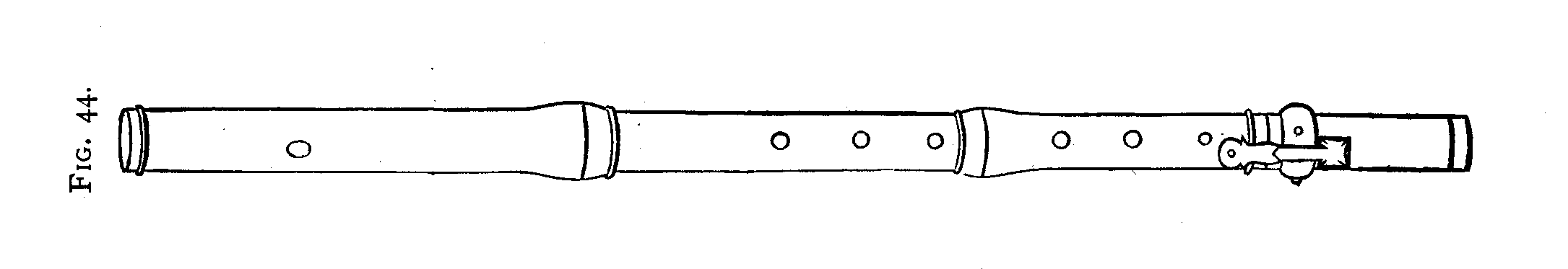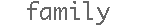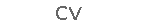Cork Position.

The position of the cork of a traverso is essential for the intonation and the tone quality. It is not straightforward where this should be and often problems simply come from a mistake in this position.

Let me first give briefly what the rules are and roughly why. Then I will further give some basic reasoning behind those simple rules.

The cork should be positioned such that d/2 and d/3 are exactly an octave, not necessarily d/1 and d/2.

Then the intonation and tone quality should be optimal. The lowest d on most (original also) traverso then is a bit too low. It should not be tried to correct this with the cork position. But it is easily corrected by embouchure.
Simplified the reason is the following. If the cork is moved 1mm. d/1 changes in the order of 1.5 cent, d/2 changes in the order of 3 cents and d/3 in the order of 6 cents. I will be more precise in the following. If we change the cork 3 mm. d/1 goes in the order of 4.5 cents, d/2 in the order of 9 cents, d/3 18 cents.

Let us consider an example (in reality the cents depend non linearly on the cork position but because the changes are small with respect to the wave length this is also practically linear).
Suppose that the cork is in the position where d/2 and d/3 are an exact octave and d/1 is e.g. -5 cents without compensating. The cork would have to move 3 mm. to make d/1 5 cents higher. But then d/2 is 10 cents higher and d/3 20 cents higher. This gives a d/2-d/3 that is 10 cents off. And on top of that d/1 is still 5 cents low with respect to d/2!

Experience shows that with many originals the rest of the intonation and the tone quality are optimal with d/2-d/3 an exact octave. This further illustrates that this is the right choice.

We could easily change the intonation of the traverso to make d/1 higher but it effects the character of the whole flute negatively.

The above rule is correct but the numbers (1.5 cent is 1 mm.) need some further consideration.
In the following I will try to be a bit more precise although an exact calculation would not be simple as will be seen. First I will look at it as if the cork position change has exactly the same effect on the sounding length.

The above more precise.

In the following we take the a/1 to be 415Hz.
Then the frequency of d1 is 415*3/4=311.25 Hz because a-d is a fifth. Let us take the speed of sound 343 m/sec. Then the wave length of d1 is 1.102 m. So d/2 is 622.5 Hz and has a wave length of 0.551 m. and d/3 is 1245 Hz and is 0.275.5 m. A shortening of the wave length of 1 mm by moving the cork position therefore is a frequency of 311.535 Hz for d/1. Translating this in equal temperament cents gives 1.6 cents (for the experts: 1200*2log(1102/1101), yes the 343 cancels)
For d/2 the same story gives 3.15 cents and for d/3 it is 6.3 cents (again for the experts Taylor development shows that because 1 mm. is small it is almost linear).
For a shift of 3 mm. this gives for d/1 4.7 cents, for d/2 9.5 cents for d/3 19 cents.

This is not correct because the mouth hole stays in the same position and the cork end is not a node. The graph on p 494 in  shows this. I am simply not able to adapt the calculation for this.

Everybody can do the practical experiment by pushing in the cork for instance 3mm. The d/1 will hardly change but d/2 will and d/3 about double.

In  we find some remarks on the change of the cork positionwhen using different joints.
Basically it says the following. The effect of a longer so lower joint should be more on the higher notes. Everything in intonation is relative. So the third d should go down more then the second. So the cork should go in because this pushes the third d more up then the second.

Hoever, the bore of the longer joint is different so this does not just simply apply.

Arthur H. Benade Fundamentals of Musical Acoustics. Dover 0-486-26484-X

Tromlitz Johann George, Ausfuehrlicher und gruendlicher Unterricht die Floete zu spielen. http://imslp.org/wiki/Ausf%C3%BChrlicher_und_gr%C3%BCndlicher_Unterricht_die_Fl%C3%B6te_zu_spielen_%28Tromlitz,_Johann_George%29

Simon Polak: Early Flutesoriginals

privatescJsHost+ "statcounter.com/counter/counter.js'>");